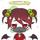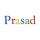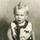## Social Question# Can you solve this reverse geometry problem?

Asked by LostInParadise (27668) April 20th, 2012

I am sure you all know how to plot points on a grid. How about going in the other direction. You are given two points on a circular piece of paper along with their coordinates. The x coordinates of the two points are different and so are the y coordinates. The scaling is the same for both x and y. Using a ruler (not just a straightedge), a compass and, if necessary, a protractor, can you draw the coordinate axes?

I think this would make a good problem for a geometry class. The problem requires no knowledge of either Euclidean geometry or algebra.

Observing members: 0Composing members: 0Here is my approach: You take the compass and draw a circle with the radius equal to the x coordinate of each point. draw the shared tangents of each circle in red. Do the same for the y values of the points and draw the tangents in blue. Each pair of perpendicularly intersecting red/blue tangents is one of the possible axis pairs.
Now I am not sure which one is the correct axis pair or if both pairs can equally be true, but it is 30 past 24 here.

ragingloli (46984)“Great Answer” (2) Flag as…@ragingloli , That was my first approach. There is another way, which does not require the use of shared tangents.

LostInParadise (27668)“Great Answer” (0) Flag as…You could also draw a circle around one of the point, the radius being y1-y2 and around the second point a circle with the radius x1-x2. the intersection between those two gives you a 3rd point with which to construct a right angled triangle. then you extend the two short sides by the smallest x/y values of the coordinates to get the axes.

ragingloli (46984)“Great Answer” (3) Flag as…I may go about finding which side the origin lies with respect to the points. You have two points with different coordinates altogether. Notice X coordinates of two points. Notice which is smaller and which is larger. The Y axis (or the origin) lies to the side to the direction given by an arrow drawn from larger X coordinate to the smaller X coordinate. Similarly, do it for Y coordinates. Now we know which side the origin is.

To go actually plot the origin, we need the scale of the plot. Measure distance between the two points with the ruler (point to point straight distance, may be a line at some angle). Find the distance between the two points by the distance formula. With both distances in hand, you can find out the scale then. Suppose, you measured the distance 4 units with ruler and calculated it to be 8 units with the distance formula, then the scale is 1 unit of the ruler equals 2 units of the figure. I guess, you can go further plotting it to the origin then.

Why did you say about the circular paper?

prasad (3854)“Great Answer” (1) Flag as…@ragingloli , Nicely done.
@prasad , The reason for the circular paper is because if the paper is rectangular, people would assume that the axes are parallel to the sides of the paper. You are correct about needing to use the distance formula for finding the scaling factor.

LostInParadise (27668)“Great Answer” (1) Flag as…It occurred to me that this can be solved with a straightedge in place of the ruler provided that all the coordinates are numbers that can be constructed with straightedge and compass , which includes rationals and all square roots of integers. . You need to know the basic properties of similar triangles.

If the two points are P1(x1, y1) and P2(x2,y2), let P3 = (x2,y1), the point constructed by @ragingloli.

To construct P3, choose an arbitrary unit measure to construct a right triangle having legs with lengths of (x2 -x1) and (y2 – y1) in terms of the selected unit. The triangle is similar to P1-P2-P3. If you mark off length P1P2 on the hypotenuse of this triangle and drop a perpendicualr, you get a triangle congruent to P1-P2-P3. Knowing the lengths P1P3 and P2P3, use @ragingloli ’ s method to construct P3.

Using similar triangles again, it is possible to get the unit length from the segment connecting P1 and P3, and then use this unit length to construct x1 and y1 to locate the origin.

LostInParadise (27668)“Great Answer” (1) Flag as…Figure the differences in x coordinates and y coordinates, and the ratio. Use a carpenter’s square so it touches both points with the numbers in the same ratio as the ratio of those differences. Now you have established which way the axes point and it is a simple matter to figure out how big the units are, if they are not inches or centimeters, and to measure to them.

SmartAZ (1802)“Great Answer” (0) Flag as…